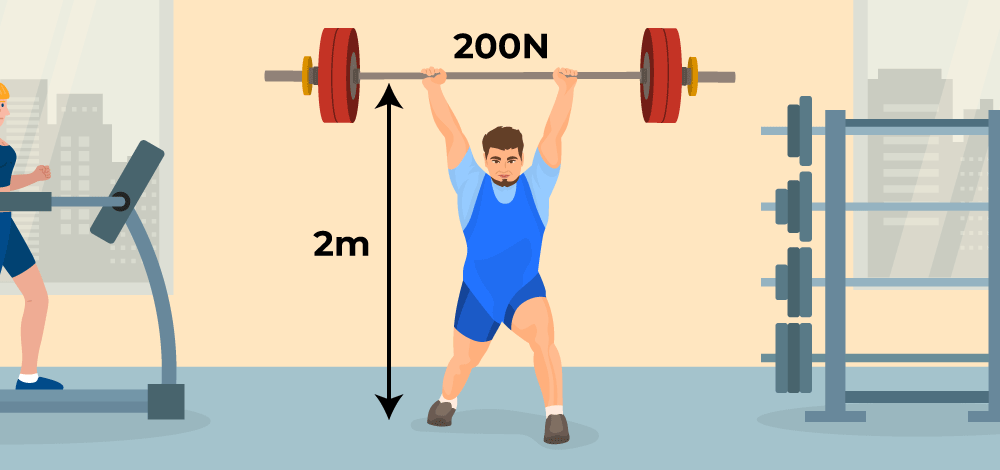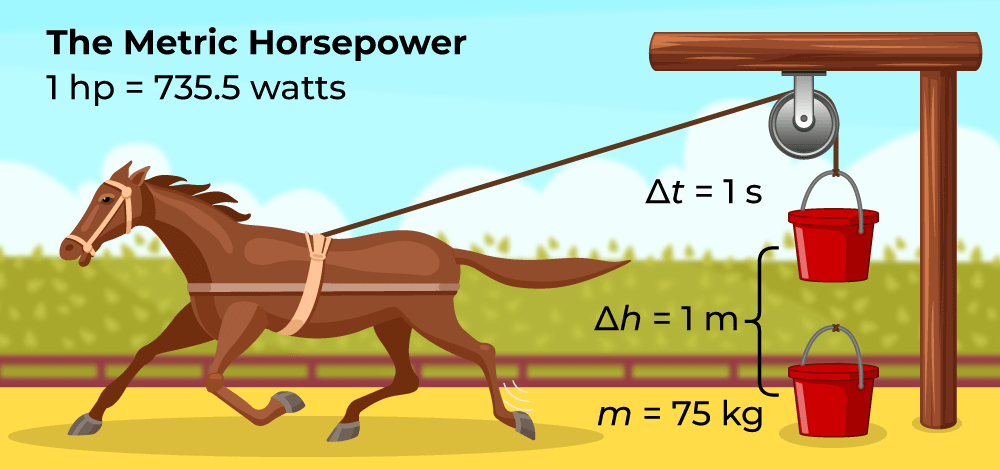GFG App
Open AppBrowser
Continue

Power in Physics is defined as the time rate of the amount of energy converted or transferred. In the SI system (or International System of Units), Watt (W) is the unit of Power. Watt is equal to one joule per second. In earlier studies, power is sometimes called Activity. Power is a scalar quantity. Power is a function of Work done, therefore if a person works at a variable rate depending on the time of day, his power will likewise vary.

## What is Power?

Power is a physical quantity that is a measure of energy transfer rate. Therefore, it can be defined as the rate at which work is done with respect to time.

Hence, Power is the amount of energy consumed per second. Power can also be defined as the quantity of force required to cause a unit displacement. Power is a scalar quantity and is denoted by P.

For example, a bulb with a higher power or wattage, like 100 W, illuminate more light than a light bulb of 10 W. Thus, this implies that more energy is illuminated by the bulb consuming more power.

The image given below depicts a man lifting a weight and showing his power. Here the power is muscular power## Power Formulas

Power is defined as the time rate of work done by the object, then, mathematically this can be given by the power formula:

P = W ⁄ t

where,
W is the Work done,
t is the Time for which work is done, and
P is the Power gain or loss.

Hence, the above relation of Power is called the work-time equation

Work done (W) by an object can be defined as the product of force and the displacement of the object, then the power formula in terms of force is given by-

Since, W = F × s

Therefore,

P = (F × s) ⁄ t

where,
F is the force required,
s is the displacement of the object,
t is the time taken.

### Other Power Formulas

The other formulas for calculating the power are discussed below,

Since, the velocity of the object, v = s / t

Then,

P = F × v

where,
F is the Force required,
v is the velocity of object.

Hence, the above relation is known as the Force-Velocity Equation

## Units of Power

• The SI unit of Power is Watt (W) whose multiples are, KW, MW, GW, and Others.

A Watt is defined as, when a body does work of one joule in one second it is called One-Watt Power.

1 Watt (W) = 1 Joule (J) / 1 Second (s)

• Another unit of power is Horsepower (hp), where 1 hp = 746 W
• Dimensional Formula for Power is [ML2T-3]

## How do We Measure Power?

Power is measured in various units and the SI unit for measuring power is the ‘watt’ represented by the symbol (W). It was named after Scottish scientist James Watt who first came up with the idea of measuring power.

Power is calculated using the formula,

P  = ΔE / Δt

where,
ΔE is the change in energy
Δt is the time taken in seconds

Power can also be measured in Joule/sec.

### What is Horsepower?

Interestingly as the name suggests horsepower does not have to do anything with horses it is the unit of measuring the power of any machine or engine. It is denoted by ‘hp’. It is mathematically equal to,

1 hp = 736 watts

Generally, the horsepower or hp is used to measure the power output of the engines of cars and bikes. The following image shows the power in Horsepower.## Average Power

Average Power is defined as the ratio of the net (Total) work done in the total amount of time. Thus average power is given as,

Average Power = Total Work Done / Total Time Taken

or

Pav = ΔW / ΔT

where,
Pav is the Average Power,
ΔW is the Total Work Done, and
ΔT is the total amount of time taken.

In case when the rate of work done by the body is uniform or constant then the average and instantaneous power become equal.

## Mechanical Power

In mechanical systems, the sum of forces and motion is known as power. Power is generally the product of a force acting on an object multiplied by its velocity, or the result of torque acting on a shaft multiplied by its angular velocity.

The time derivative of work is another way to define mechanical power. Hence, the mechanical power is given by.

Mechanical Power = Force × velocity

or

Pm = F × v

## Electrical Power

The rate at which energy is changed from the electrical energy of moving charges to another form, expressed as a rate, per unit of time, is known as electric power.

Mathematically, electric power is defined as the product voltage and the current flowing, given as:

P = V × I

According to Ohm’s Law. V = I × R, therefore,

P = I2 × R

or

P = V2 / R

where,
P is the electric power,
I is the current flowing,
R is the resistance and
V is the voltage.

## Calculating Power and Energy Consumption

The power and energy consumption are measured by a formula. According to this, multiplying the number of power units consumed within the period over which it has been consumed gives it energy consumption.

For more detail, Commercial Unit of Electrical Energy

Hence, the energy consumption formula or the power consumption formula can be stated as:

E = P × (t/1000)

where,

• E is the Energy consumed or power consumed
• P is the power and
• t is the time over which the power or energy was consumed.

Energy consumed or power consumed is generally measured in Joules or kilowatt-hours (kWh).

Also, Check

## Solved Examples on Power

Example 1: A boy pushes a box of 20 kg up to a distance of 5 m for 10 seconds. Calculate the power delivered to the box.

Solution:

Given,

Mass of the box, m = 20 kg

Displacement covered, d = 5 m

Time of displacement, t = 10 s

Weight of the box, F = mg = 20 ×10 N = 200 N

Work done by the boy, W = F d = 200 N ×5 J = 1000 J

Power delivered, P = W ⁄ t = 1000 / (10 J/s) = 100 J/s

Hence, power delivered to the box is 100 J/s.

Example 2: A pump is required to lift 500 kg of water per minute from an 8 m deep well and eject it with a speed of 25 m/s. Calculate the power of the pump.

Solution:

Given,

Mass of the water, m = 500 kg

Height covered, h = 8 m

Eject velocity of water, v = 25 m/s

Delivery time, t = 1 min = 60 s

Total energy is converted into work,

W = E = m g h+(1/2) m v2

= (500×10×8)+(500×25×25)/2

= (40000+156250) J

=196250 J

Power delivered,

P = W / t

= 196250 / 60

= 3271 W

Hence, power delivered by pump is 3271 W.

Example 3: An elevator is designed to lift a load of 500 kg through 5 floors of a building averaging 3 m per floor in 5 seconds. Calculate the power of the elevator.

Solution:

Given:

Mass of the load, m = 500 kg

Total height covered, h = 5 × 3 m =15 m

Time taken, t = 5 s

Power delivered by elevator,

P = W ⁄ t = mgh ⁄ t

= (500 × 10 × 15) / 5 W

= 15000 W

= 1.5×104 W

Hence, power of the elevator is 1.5×104 W.

Example 4: A force of 5 N is required to move a body on a frictional floor with a constant velocity of 5 m/s. Find the power generated by the force.

Solution:

Given:

Velocity of body, v = 5 m/s

Force required to maintain the velocity, F = 5 N

Power generated,

P = 5 × 5 W

= 25 W

Hence, power generated by the force is 25 W.

## FAQs on Power

### Q1: What is Power in Physics?

Power is the amount of energy consumed per second. Power can also be defined as the quantity of force required to cause a unit displacement.

### Q2: What is the dimension of power in physics?

Power is defined as the rate of work done. The dimension formula of Power is [ML2T-3].

### Q3: How to calculate power in physics?

Power can be determined by the formula,

P = W ⁄ t

where
W is the Work done,
t is the Time for which work is done, and
P is the Power gain or loss.

### Q4: What is the difference between Power and Energy?

Energy is defined as the ability to do some physical work, e.g playing, jumping, etc. However, power is defined as the rate at which the energy is transferred, or the work is completed.